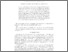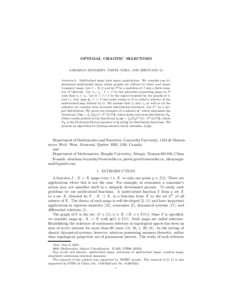Title:

# Optimal chaotic selectors

Boyarsky, Abraham, Góra, Paweł and Li, Zhenyang (2015) Optimal chaotic selectors. Dynamical Systems . pp. 1-17. ISSN 1468-9367Preview Text (application/pdf) Optimal_Chaotic_Selectors14.pdf - Accepted Version Available under License Spectrum Terms of Access. 303kB

Official URL: http://dx.doi.org/10.1080/14689367.2015.1006585

## Abstract

Multivalued maps have many applications. We consider one dimensional multivalued maps whose graphs are defined by lower and upper boundary maps. Let $I=[0,1]$ and let $\mathcal P$ be a partition of $I$ into a finite number of intervals. Let $\ltau ,$ $\utau :I\rightarrow I$ be two piecewise expanding maps on $\mathcal P$ such that $\ltau\le\utau$. Let $G$ $\subset I\times I$ be the region bounded by the graphs of $\ltau$ and $\utau.$ Any map $\etta :I\rightarrow I$ that takes values in $G$ is called a selector of the multivalued map defined by $G.$ We assume that $\ltau$ and $\utau$ as well as all the selectors we consider have invariant distribution
functions. Let $F^{\ast}$ be a target distribution. We
prove the existence of a selector $\etta ^{\ast }$ which minimizes the functional $J(\etta )=\int_{I}(F_{\etta }(t)-F^{\ast }(t))^{2}dt$, where $\etta$ has invariant distribution $F_{\etta }$. Other results pertain to the
functional $J_{1}(\etta )=\int_{I}(P_{\etta }F^{\ast }(t)-F^{\ast }(t))^{2}dt$, where $P_{\etta }$ is the Frobenius-Perron operator of $\etta$ acting on
distribution functions. We present an algorithm for finding selectors which minimize $J_{1}(\etta )$.

Divisions: Concordia University > Faculty of Arts and Science > Mathematics and Statistics Article Yes Boyarsky, Abraham and Góra, Paweł and Li, Zhenyang Dynamical Systems 2015 10.1080/14689367.2015.1006585 980101 PAWEL GORA 25 Jun 2015 20:18 18 Jan 2018 17:50
All items in Spectrum are protected by copyright, with all rights reserved. The use of items is governed by Spectrum's terms of access.

Repository Staff Only: item control pageResearch related to the current document (at the CORE website)
Back to top